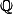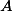# Martin Orr's Blog

## The Chowla-Selberg formula

Posted by Martin Orr on Monday, 28 December 2015 at 20:00

The Chowla-Selberg formula is an equation which expresses the periods of CM elliptic curves in terms of values of the gamma function at rational arguments. Colmez conjectured a generalisation of the Chowla-Selberg formula to higher-dimensional CM abelian varieties, and an averaged version of Colmez's conjecture was recently proved by Andreatta, Goren, Howard and Madapusi Pera and independently by Yuan and Zhang. This has been much talked about in the world of abelian varieties, because Tsimerman used the averaged Colmez conjecture to complete the proof of the André-Oort conjecture for.

I thought that rather than looking directly at the Colmez conjecture, it would be good to start with the simpler Chowla-Selberg formula i.e. the elliptic curves case. In this post I will talk about a couple of ways of stating the formula, in terms of periods of CM elliptic curves or in terms of Faltings heights.

## The Faltings height and normed modules

Posted by Martin Orr on Saturday, 31 December 2011 at 15:31

In this post I shall give the definition of the Faltings height of an abelian variety over any number field. Last time we did this overonly, and we used two properties of: the integers are a PID and there is only one archimedean place. To do things more generally, we will introduce the technology of normed modules and their degrees.

## The Faltings height of an abelian variety over the rationals

Posted by Martin Orr on Thursday, 17 November 2011 at 15:58

The Faltings height is a real number attached to an abelian variety (defined over a number field), which is at the centre of Faltings' proof of Finiteness Theorem I. In this post all I will do is define the Faltings height of an abelian variety over, as already this requires a lot of preliminaries on cotangent and canonical sheaves of schemes. Further complications arise over other base fields, which I will discuss next time.

For an abelian varietyover, the Faltings height is the (logarithm of the) volume ofas a complex manifold with respect to a particular volume form, chosen using the-structure of. The preliminaries are needed in order to choose the volume form.

Faltings' proof of Finiteness I proceeds by showing that for any fixed number field, there are finitely many abelian varieties of bounded Faltings height. This is done by showing that the Faltings height is not far away from the classical height of a point representing the abelian variety in the moduli space. Then he shows that the Faltings height is bounded within an isogeny class. Both of these parts are difficult.

## Shafarevich and Siegel's theorems

Posted by Martin Orr on Friday, 07 October 2011 at 09:00

In this post I will prove the Shafarevich conjecture for elliptic curves (also called Shafarevich's theorem). The proof is by reducing it to the finiteness of the number of solutions of a certain Diophantine equation, and then applying Siegel's theorem on integral points on curves.

Shafarevich's Theorem. Letbe a number field anda finite set of places of. Then there are only finitely many isomorphism classes of elliptic curves overwith good reduction outside.

Siegel's Theorem. Letbe a number field anda finite set of places of. An absolutely irreducible affine curveoverof genus at leasthas only finitely many-integral points.

Since the reduction of Shafarevich's theorem to Siegel's theorem is short, and Siegel's theorem is of independent interest, most of the post will be about Siegel's theorem.

## Finiteness theorems for abelian varieties

Posted by Martin Orr on Monday, 19 September 2011 at 16:34

Faltings famously proved the Mordell, Shafarevich and Tate conjectures in 1983. In this post I will discuss the relationships between the Tate and Shafarevich conjectures and some other finiteness theorems for abelian varieties.

Everything which I call a conjecture in this post is known to be true: they all follow from Finiteness Theorem I. Proving Finiteness Theorem I was the bulk of Faltings' work, but I am not going to talk about that today.

Finiteness Theorem I. Given a number fieldand an abelian varietydefined over, there are only finitely many isomorphism classes of abelian varieties defined overand isogenous to.